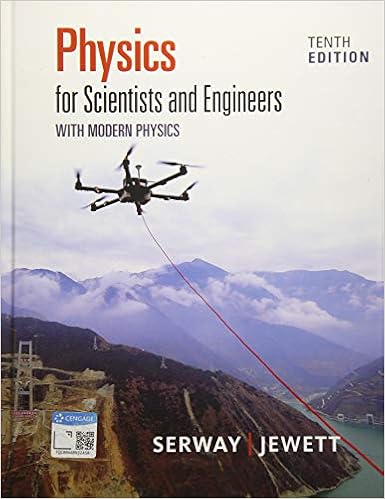K 1 2 i tot ω 2 1 2 1 12 ml 2 m 3 l 2 2 ω 2 1 12 ml

• Test Prep
• 9
• 100% (3) 3 out of 3 people found this document helpful

This preview shows page 8 - 9 out of 9 pages.

We have textbook solutions for you!
The document you are viewing contains questions related to this textbook.The document you are viewing contains questions related to this textbook.
Chapter 2 / Exercise 2.1
Physics for Scientists and Engineers with Modern Physics
Jewett/SerwayExpert Verified
K = 1 2 I tot ω 2 = 1 2 1 12 Ml 2 + M 3 ! " # \$ % & l 2 ! " # \$ % & 2 ' ( ) ) * + , , ω 2 = 1 12 Ml 2 ω 2 = 21.3 J (before explosion) K ' = 1 2 I rod ω ' 2 + 1 2 M 3 ! " # \$ % & v p 2 = 1 2 1 12 Ml 2 ! " # \$ % & ω ' 2 + 1 2 M 3 ! " # \$ % & v p 2 (after explosion) Δ K = K ' K = M 2 1 12 l 2 ω ' 2 + 1 3 v p 2 1 6 l 2 ω 2 ! " # \$ % & = 3.0 J
We have textbook solutions for you!
The document you are viewing contains questions related to this textbook.The document you are viewing contains questions related to this textbook.
Chapter 2 / Exercise 2.1
Physics for Scientists and Engineers with Modern Physics
Jewett/SerwayExpert Verified
Problem 5 (27 pts.): A block of mass m 1 rests on a frictionless inclined plane at a height above a flat horizontal plane. This mass is released and slides down the incline, eventually colliding elastically with a second block at rest at x 0 with mass m 2 , with m 1 = 3 m 2 /4. Immediately after the elastic collision, block number 2 moves to the right with velocity v 2f . It encounters a region that has friction with a coefficient of kinetic friction of μ k , traveling a distance d before coming to rest. Answer the following questions about these two blocks. a) ( 7 pts.) Determine an equation for v 2f immediately after the collision (using m 2 , h , and h = g ) 2 b) ( 7 pts.) Determine an equation for d (in terms of m 2 , h , μ k , and g ), the distance that block 2 c) ( 6 pts.) What is the magnitude (equation) and sign of the work done by the frictional force on block 2? d) ( 7 pts. ) What is the velocity v f of block 1 after the collision (in terms of m 2 , h , and g ). Use vector notation. 1 1
•••